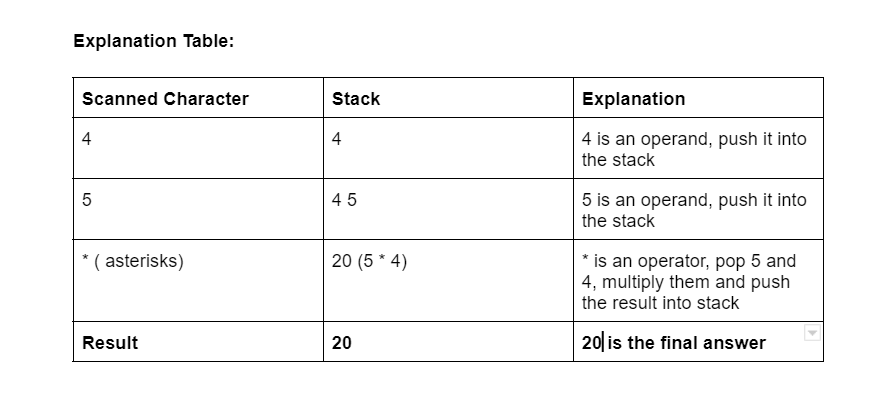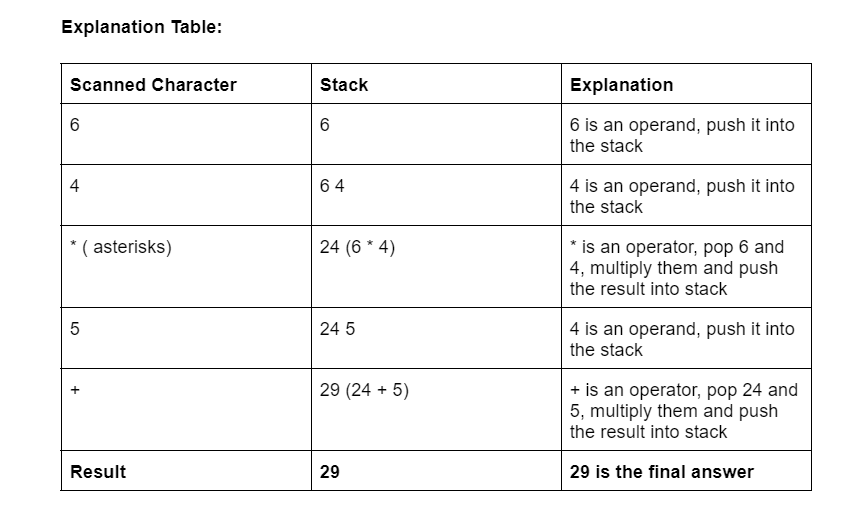# Evaluation of Prefix expression

Problem Statement: Evaluation of prefix expression step by step.

Examples:

```Example 1:
Input: * 5 4
Output: 20
Explanation: Refer to Explanation table no.1 (Below)

Example 2:

Input: + 5 * 4 6
Output: 29
Explanation: Refer Explanation table no. 2 (Below)
```

### Solution

Disclaimer: Don’t jump directly to the solution, try it out yourself first.

### What is the prefix Notation/Expression?

In prefix expression, The operator is written before the operands.

Eg: The infix Expression A+B can be written as in the prefix form as +AB

• The prefix expression as the name suggests has the operator placed before the operand is specified.
• It is of the form <operator><operand><operand>.
• It works entirely in the same manner as the postfix expression.
• While evaluating a prefix expression, the operators are applied to the operands immediately on the right of the operator.
• For evaluation, we evaluate it from left to right. Prefix expressions are also called polish notation.

This article will be based on how to evaluate prefix expressions

For more information related to infix, prefix, and postfix Expression/NotationsRefer: Infix, Prefix, and Postfix Introduction

Approach

• Initialise a pointer ptr at the end of the expression
• If the character at the pointer ptr is an operand push it into the stack
• If the character at pointer ptr is an operator then pop the top two elements from the stack. Perform the calculations on these elements according to the operator, and push the result back into the stack
• Decrement pointer ptr by 1 and repeat step 2 & step 3 until the entire expression is not scanned
• The Result is stored at the top of the Stack Print / Return it
```Explanation table no.1
Expression: * 4 5
Output: 20
``````Explanation table no.2
Expression: + 5 * 4 6
Output: 29
```Code:

## C++ Code

``````#include <bits/stdc++.h>

using namespace std;

bool chk(char c) {
// If the character is a digit then it must
// be an operand
return isdigit(c);
}

double prefix(string s) {
stack < double > Stack;

for (int j = s.size() - 1; j >= 0; j--) {

// Push operand to Stack
// To convert exprsn[j] to digit subtract
// '0' from exprsn[j].
if (chk(s[j]))
Stack.push(s[j] - '0');

else {

// Operator encountered
// Pop two elements from Stack
double o1 = Stack.top();
Stack.pop();
double o2 = Stack.top();
Stack.pop();

// Use switch case to operate on o1
// and o2 and perform o1 O o2.
switch (s[j]) {
case '+':
Stack.push(o1 + o2);
break;
case '-':
Stack.push(o1 - o2);
break;
case '*':
Stack.push(o1 * o2);
break;
case '/':
Stack.push(o1 / o2);
break;
}
}
}

return Stack.top();
}

int main() {
string s = "+5*46"; // Expression string
cout << prefix(s) << endl;
return 0;
}``````

Output: 29

Time Complexity: O(N)

Space Complexity: O(N)

## Java Code

``````import java.io.*;
import java.util.*;

class TUF {

static Boolean chk(char c) {
// If the character is a digit
// then it must be an operand
if (c >= 48 && c <= 57)
return true;
else
return false;
}

static double evaluatePrefix(String s) {
Stack < Double > Stack = new Stack < Double > ();

for (int j = s.length() - 1; j >= 0; j--) {

// Push operand to Stack
// To convert exprsn[j] to digit subtract
// '0' from exprsn[j].
if (chk(s.charAt(j)))
Stack.push((double)(s.charAt(j) - 48));

else {

// Operator encountered
// Pop two elements from Stack
double o1 = Stack.peek();
Stack.pop();
double o2 = Stack.peek();
Stack.pop();

// Use switch case to operate on o1
// and o2 and perform o1 O o2.
switch (s.charAt(j)) {
case '+':
Stack.push(o1 + o2);
break;
case '-':
Stack.push(o1 - o2);
break;
case '*':
Stack.push(o1 * o2);
break;
case '/':
Stack.push(o1 / o2);
break;
}
}
}

return Stack.peek();
}

public static void main(String[] args) {
String s = "+5*46";
System.out.println(evaluatePrefix(s));
}
}``````

Output: 29

Time Complexity: O(N)

Space Complexity: O(N)

## Python Code

``````def is_operand(c):
"""
Return True if the given character is an operand
"""
return c.isdigit()

def evaluate(expression):
"""
Evaluate a given expression in prefix notation.
Asserts that the given expression is valid.
"""
stack = []

# iterate over the string in reverse order
for c in expression[::-1]:

# push operand to stack
if is_operand(c):
stack.append(int(c))

else:
# pop values from stack can calculate the result
# push the result onto the stack again
o1 = stack.pop()
o2 = stack.pop()

if c == '+':
stack.append(o1 + o2)

elif c == '-':
stack.append(o1 - o2)

elif c == '*':
stack.append(o1 * o2)

elif c == '/':
stack.append(o1 / o2)

return stack.pop()

if __name__ == "__main__":
test_expression = "+5*46"
print(evaluate(test_expression))
``````

Output: 29

Time Complexity: O(N)

Space Complexity: O(N)

Special thanks to Abhishek Yadav for contributing to this article on takeUforward. If you also wish to share your knowledge with the takeUforward fam, please check out this article